## Angular Momentum

• Angular momentum is the rotational analogue of linear momentum in Newtonian physics.

The angular momentum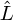of a solid body is the product of its moment of inertia I and angular velocity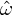.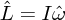In a closed system angular momentum is conserved. Curiously, angular momentum is a vector quantity, and points in the same direction as the angular velocity of the object.

The angular momentum of a system of N particles is just the vector summation of all of its constituents.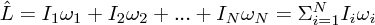The angular momentumof a point particle of mass m, moving with velocity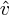, at a distance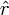, from some reference point is: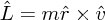where the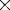is the vector cross product. The direction of the vector is given by the right hand rule - by holding the fingers in the direction ofand sweeping them towards, the thumb dictates the direction of the resultant vector.